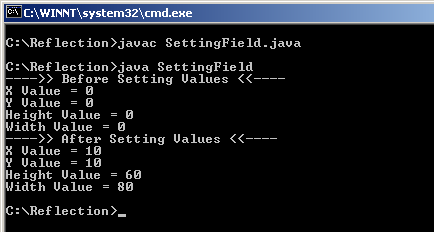Tutorials

# Setting Fields Information of a class using Reflection

As in our previous example we have seen that we can get field values of a class by using the Field class. Now we can also set different field values of that class by using set() method.

As in our previous example we have seen that we can get field values of a class by using the Field class. Now we can also set different field values of that class by using set() method.

# Setting Fields Information of a class using Reflection

As in our previous example we have seen that we can get field values of a class by using the Field class. Now we can also set different field values of that class by using set() method.

In our class SettingField we have created an object of "Rectangle" and we can get fields of that object by using getField() method and we can retrieve values of that fields by get() method. Now we can set fields value by using set() methods.

 ```     xfield.setInt(rect,new Integer(10));   yfield.setInt(rect,new Integer(10));   heightField.setInt(rect,new Integer(60));   widthField.setInt(rect,new Integer(80));```

Above lines of code sets x-axis, y-axis, height, width field values. Since we are setting integer values to its field so I have used setInt() method. Here is the example code for SettingField class :

SettingField.java

 ``` import java.awt.*; import java.lang.reflect.*; public class SettingField{   public static void main(String[] args) throws Exception{   Rectangle rect=new Rectangle();   Field xfield = rect.getClass().getField("x");   Field yfield = rect.getClass().getField("y");   Field heightField = rect.getClass().getField("height");   Field widthField = rect.getClass().getField("width");   System.out.println("---->> Before Setting Values <<----");   System.out.println("X Value = "+ xfield.getInt(rect));   System.out.println("Y Value = "+ yfield.getInt(rect));   System.out.println("Height Value = "+ heightField.getInt(rect));   System.out.println("Width Value = "+ widthField.getInt(rect));   xfield.setInt(rect,new Integer(10));   yfield.setInt(rect,new Integer(10));   heightField.setInt(rect,new Integer(60));   widthField.setInt(rect,new Integer(80));   System.out.println("---->> After Setting Values <<----");   System.out.println("X Value = "+ xfield.getInt(rect));   System.out.println("Y Value = "+ yfield.getInt(rect));   System.out.println("Height Value = "+ heightField.getInt(rect));   System.out.println("Width Value = "+ widthField.getInt(rect));  } }```

Output: